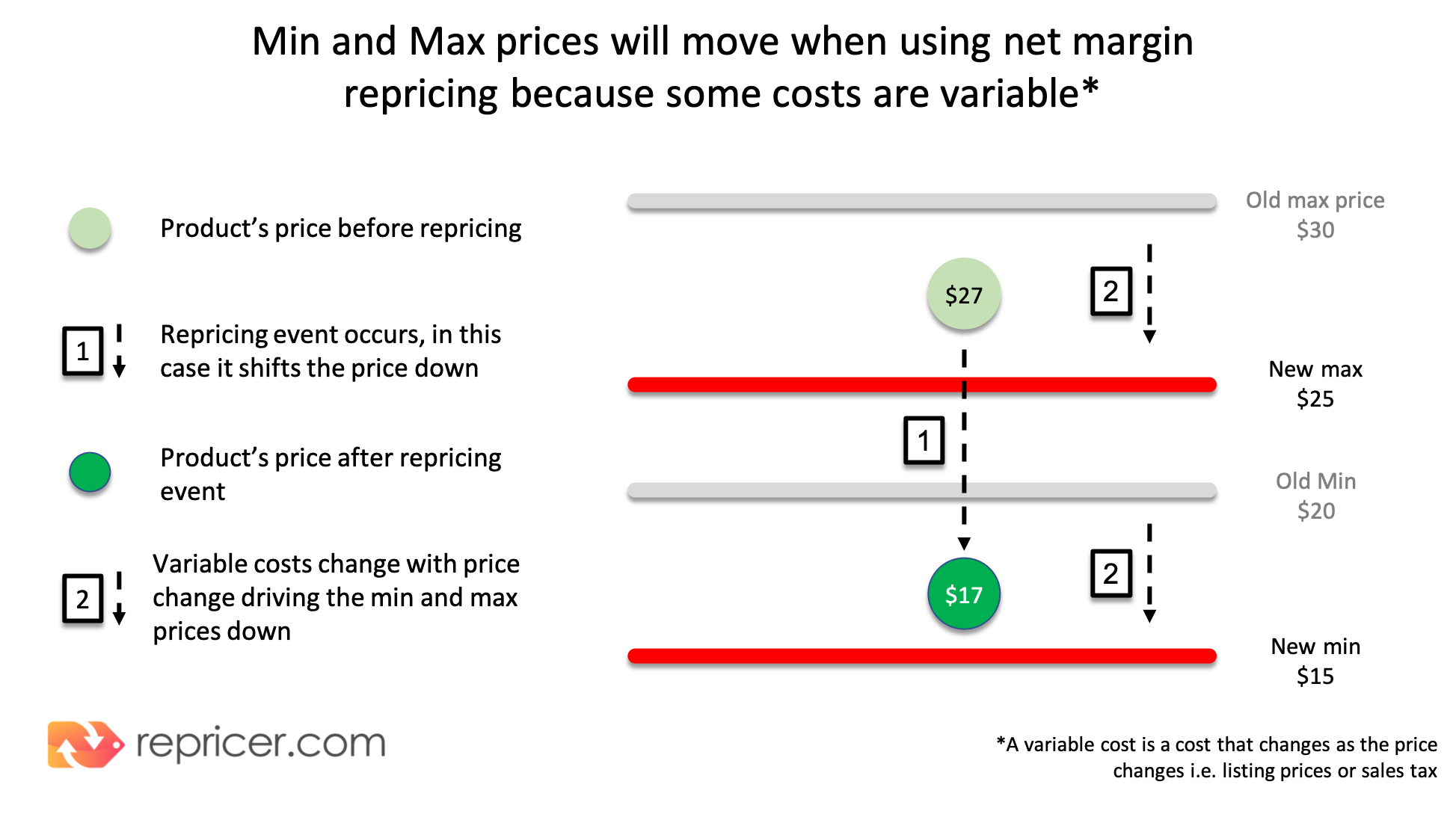Net Margin is a measure of profitability. It is calculated by finding the net profit as a percentage of revenue. If you sold an item for \$100.00 and the total cost of the item to you was \$60.00 your net profit is \$40.00. We can also say that your Net Margin was 40% (\$40/\$100). In this article we'll explain a bit more about Net Margin and how it works in Repricer.

Note: At the moment Net Margin Repricing is only available for Amazon but will be added to eBay in the future. Let us know if this is a must have feature for you by reaching out at suggestion@repricer.com

## Understanding how Net Margin Repricing works

In this section, we'll have a deeper look at how Net Margin Repricing works in your Repricer account.

• Why are my costs changing?: Some of your selling costs, such as listing fees and VAT or sales tax, are variable; they change as your selling price changes. As a result, when the Repricer changes your price, your costs will also change.

• How does net margin repricing affect my max and min?: When you’re using net margin repricing you’re deciding to set your minimum and maximum against costs that are changing. Therefore, your max and min will change every time the Repricer changes your price. However, because you’ve input a minimum net margin you’ll always make a profit.• Why your profit is protected despite your changing costs?: While some of your costs are variable (i.e. listing fees and VAT or sales tax) others are fixed, that is they remain constant regardless of what your price is. Unit cost, Shipping cost, and Pickpack cost are examples of fixed costs. Your minimum cost will never go beneath your total fixed costs + your variable costs + the minimum margin you’ve input.

• Why am I unable to enter my desired Net Margin: Net Margin % is the percentage of your selling price that is profit. This means that some values of Net Margin % are mathematically impossible. For example, you can't have a Net Margin % of 100%. In order to avoid selling at the wrong price due to incorrect inputs, you cannot input a margin of higher than 65% as your minimum.

## How do you calculate variable costs?

Within Repricer you can change where VAT applies as part of your Net Margin and Profitability calculations. For the below example we use our default approach which is to apply VAT as part of your final product sale price. Learn about the other options available here. In the example below, we’ll use a notional price of \$24 to show how your variable costs of VAT (or sales tax) and sellers fees are calculated.

### Calculating VAT

Selling Price: \$24
Vat (or sales tax) rate: 20%

It’s important to understand that the selling price is the Price Before VAT + 20% of the Price before VAT. Therefore, it would be faulty logic to say that the Vat is 20% of \$24 or \$4.80. Instead, we should note that the VAT rate has already been factored into \$24 selling price. In this sense, our Selling Price of \$24 is 120% of our Price Before VAT.

Let’s start by finding out what 1% of the Price Before VAT is. We can do so, by dividing the selling price by 120%. So, \$24/120 = \$0.20 (This is 1% of the Price Before VAT)

We can then multiply 1% of the Price Before VAT by 100 to find the total Price Before VAT. So, \$0.20 dollars * 100 = \$20 (This is the Price Before VAT)

And next, we’ll subtract the Price Before VAT from the Selling Price to find the Vat in \$. So, \$24 - \$20 = \$4 (VAT in \$)

### Results

Selling Price: \$24
Vat rate: 20%
Vat in \$: 4
Price Before VAT: \$20

### Calculating the selling fee

Amazon's selling fee (generally 15%) is calculated on the total selling price, that is the price including VAT. Therefore the calculation will be identical to that of VAT, except that we'll substitute the 20% VAT rate for 15% selling fee.

Selling Price: \$24
Selling fee %: 15%
Selling fee: \$3.60

### Putting it together

The variable costs at a \$24 selling price will be \$7.60. Bear in mind, that the price is fluid, and therefore, with net margin Repricing these Min and Max numbers will change with the price.